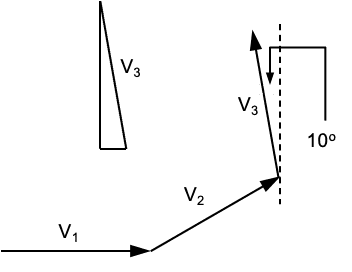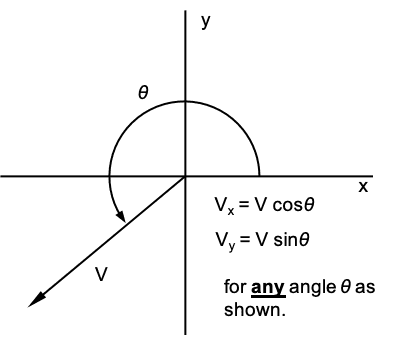# Trivial Vector Problem

• guyvsdcsniper
In summary, In Tom's sketch, V3x=-sin10o and V3y=cos10o make the most sense as shown in his new attached work.f

#### guyvsdcsniper

Homework Statement
An ant walks on a piece of graph paper straight along the x axis a distance of 10.0 cm in 2.00 s. It then turns left 30.0° and walks in a straight line another 10.0 cm in 1.80 s. Finally, it turns another 70.0° to the left and walks another 10.0 cm in 1.55 s. Determine (a) the x and y components of the ant’s average velocity, and (b) its magnitude and direction.
Relevant Equations
N/A
I am having trouble with finding the x and y components of V3 . According to various different websites the correct way to find the components of V3 is
Vx=10*cos(100) and Vy=10*sin(100). I can see where the 100 comes from, the previous vector was already traveling 30 degrees and now V3 swung out an additional 70 degrees.

The way the angle is in this triangle i sketched for V3, the opposite side of this angle presents the length of the x component. The opposite side is traveling in the X axis. Likewise, from this angle the adjacent side is traveling up which in opinion is the length of the y component.

So from my perspective to find the x and y component I should solve for
Vx=10*Sin(100) and Vy=10Cos(100).

Could some help me understand if and why I am viewing this wrong?

Work is attached in PDF

#### Attachments

• Scan Jan 30, 2021.pdf
199.6 KB · Views: 114
Vector V3 only deviates 10° from vertical; therefore, its x and y components should refer to that angle: V3x << V3y

•guyvsdcsniper
##V_{3x}=10\cos(100^o) =-10\sin(10^o)##
##V_{3y}=10\sin(100^o) =10\cos(10^o)##
Take your pick. You can always write the x-component as ##V_x=V\cos\theta## as long as ##\theta## is measured counterclockwise from the x-axis. Then you don't have to worry about right triangles. It's OK to have angles greater than 90o, they automatically take care of the negative signs. Needless to say, with this angle convention the y-component is always ##V_y=V\sin\theta.##

•Lnewqban and guyvsdcsniper
##V_{3x}=10\cos(100^o) =-10\sin(10^o)##
##V_{3y}=10\sin(100^o) =10\cos(10^o)##
Take your pick. You can always write the x-component as ##V_x=V\cos\theta## as long as ##\theta## is measured counterclockwise from the x-axis. Then you don't have to worry about right triangles. It's OK to have angles greater than 90o, they automatically take care of the negative signs. Needless to say, with this angle convention the y-component is always ##V_y=V\sin\theta.##
I really appreciate your response and it helps me understand a lot better.

I think my problem was with the way I was drawing the triangle. In my original attachment I don't think i sketched the triangle for V3 properly. I really only sketched a 70 degree triangle and it looks like the angle is pointing upward giving the impression that the x-component is on the opposite side of the triangle.

I think I properly drew the sketch of the 100 degree triangle which kinda makes it more clear on why Vy=Vsin θ and like wise for the x-component Vx=Vcos θ.

Do you agree with my logic? Does my new sketched work make more sense?

#### Attachments

• Scan Jan 30, 2021 (2) 2.pdf
177.6 KB · Views: 120
Do you agree with my logic? Does my new sketched work make more sense?
For some reason, that red dotted line of your last diagram makes me believe that it could mislead you.
The proper way to visualize these problems is by focussing on what the projection of the moving object on each axis is doing.

For example, imagine that you could stand on the x-y plane (graph paper) and that a light is projected between the moving object (ant) and your eyes.
When you are standing South of the x-axis, looking only at the projected shadow of our ant on the x-axis, you will see it moving only in the following ways:
1) From repose, 10.00 cm left to right in 2.00 seconds.
2) Then, 8.66 cm left to right in 1.80 seconds.
3) Then, 1.74 cm right to left in the last 1.55 seconds.

Using that technique, you could have an additional way to verify whether or not your numerical calculations make sense.

I think my problem was with the way I was drawing the triangle.
What triangle? Shown below is a diagram drawn to scale. The only right triangle relevant to V3 is shown as an inset where V3 is the hypotenuse. The angles opposite to the right sides are 10o and 80o. It's obvious which is which. Clearly
V3x = -V3cos80o = -V3sin10o
V3y = V3cos10o = V3sin80oLast edited:
•Delta2 and Lnewqban
What triangle? Shown below is a diagram drawn to scale. The only right triangle relevant to V3 is shown as an inset where V3 is the hypotenuse. The angles opposite to the right sides are 10o and 80o. It's obvious which is which. Clearly
V3x = -V3cos80o = -V3sin10o
V3y = V3cos10o = V3sin80o

View attachment 277202

Ok I worked it out again to try to understand. I believe using V3x = -sin10o and V3y = cos10o makes the most sense to me as shown in my new attached work. Could you help me visualize V3x=10cos(100) and V3y=10sin(100)?

#### Attachments

• Scan Jan 31, 2021.pdf
150 KB · Views: 103
Could you help me visualize V3x=10cos(100) and V3y=10sin(100)?
See picture. For 0 ≤ θ ≤ 2π, the tip of vector V traces a circle which is the unit circle scaled by the magnitude V. In this particular example V = 10 cm and θ = 30o + 70o = 100o.•Delta2, Lnewqban and guyvsdcsniper
See picture. For 0 ≤ θ ≤ 2π, the tip of vector V traces a circle which is the unit circle scaled by the magnitude V. In this particular example V = 10 cm and θ = 30o + 70o = 100o.

View attachment 277218
This makes sense to me now. I appreciate your help again.

•Delta2, Lnewqban and kuruman